# Lattice Multiplication Method

Lattice Multiplication Method• In lattice multiplication we multiply each   of the two numbers together and write these answers in the corresponding boxes.
• Each box is split diagonally.
• Each answer is split with the units to the bottom right of the diagonal and the tens to the top left.
• We move through the lattice grid to fill out all of the boxes in this way.
• Once all boxes are complete, we add up the numbers in each diagonal.
• We start at the right hand side of the grid and add the diagonals, from right to left, writing each total on the outside of the grid, in line with the diagonals.
• Finally, these totals are read from left to right and form the digits of our answer.
Multiply the digits of each number and write the answer in the corresponding box.• 86 and 67 are both   and so the lattice is 2 columns along and 2 rows down.
• We draw diagonals down from the top right, through the corners of the lattice and out of the grid.
• We multiply each digit and write the answer in the corresponding box, with the tens and units either side of the diagonal line.
• 8 x 6 = 48.
• 6 x 6 = 36.
• 8 x 7 = 56.
• 6 x 7 = 42.
• We now add the numbers in each diagonal from right to left.
• If we have any tens, we carry them to add to the next diagonal on the left.
• In the rightmost lattice diagonal we have ‘2’.
• 6 + 4 + 6 = ’16’. We write the ‘6’ but carry the ‘1’ to add to the next diagonal.
• 5 + 8 + 3 + the 1 that we just carried = ’17’. We write the ‘7’ and carry the ‘1’.
• Finally, we have 4 + the 1 that we just carried = ‘5’.
• The totals of ‘5’, ‘7’, ‘6’ and ‘2’ form our answer when read from left to right.
• 86 x 67 = 5762.# How to do the Lattice Multiplication Method

Lattice multiplication is an alternative multiplication method to long multiplication or the grid method. Lattice multiplication is used to work out the multiplication of larger numbers.

We will introduce lattice multiplication by looking at a simple example to understand how to lay out the working out.

We will consider the example of ‘3 x 4’ using lattice multiplication and we begin by drawing a square. We then draw a diagonal line from the top right corner to the opposite corner in the bottom left.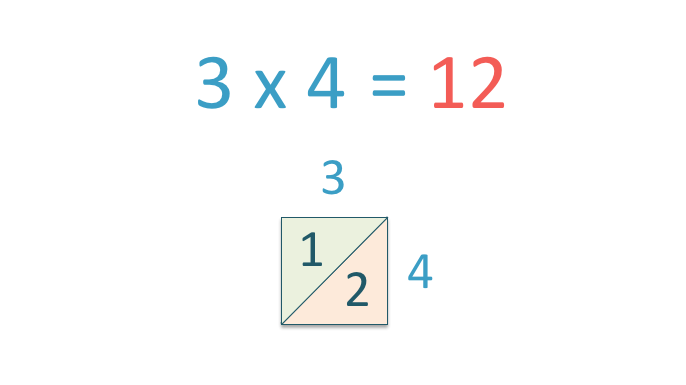3 x 4 = 12

We always write the units (or ones) of the answer to the bottom right of the diagonal line. So, we write ‘2’ in the orange shaded triangle.

We always write any tens of the answer in to the top left of the diagonal. So in this example, we write ‘1’ in the green shaded triangle shown.

If there are no tens in the answer, we write a zero to the left of the diagonal.

This is how we represent the number 12 in a lattice.

We can use this lattice structure to help us to multiply two 2-digit numbers.

Lattice Multiplication Example 1: 42 x 35

We begin by arranging the digits of 42 and 35 as shown in the image below, one number written on the top of the grid and the other number written on the right of the grid.

Each digit is lined up with its own box.Next, we multiply each of the digits of 42 by each of the digits of 35. We write the answer to each multiplication in the corresponding square.

1) 4 x 3 = 12

2) 2 x 3 = 6

3) 4 x 5 = 20

4) 2 x 5 = 10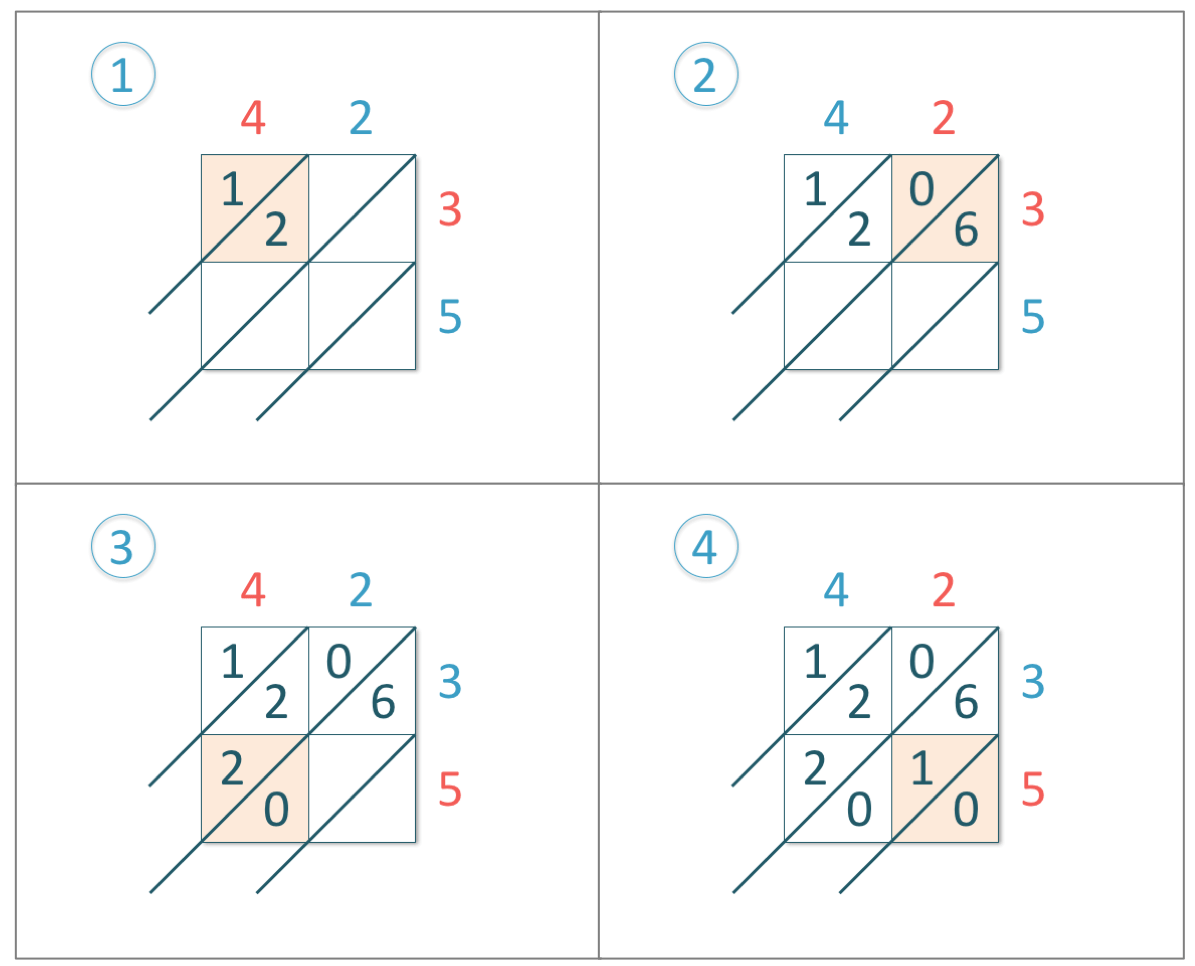Notice that when we multiplied 2 x 3 to make 6, we still wrote a ‘0’ to the left of the diagonal line.

This is so that we know that we have worked out this multiplication already, whereas if we left a blank space, we might think that we have made a mistake or missed it out by mistake.

Once we have multiplied all of the digits and filled every box in the grid, we add the digits that are in each diagonal.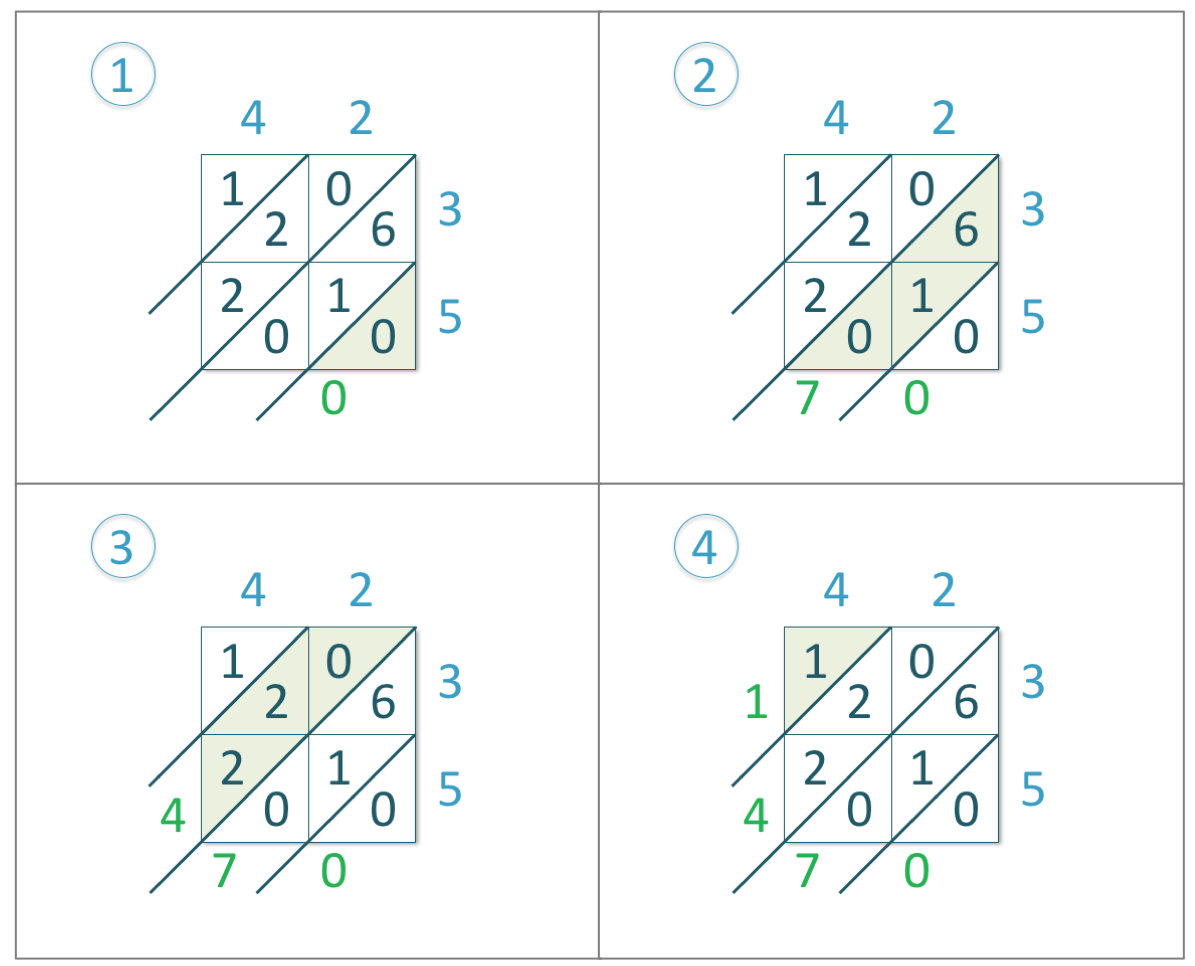We add the numbers in each diagonal starting with the bottom right diagonal and moving left.

1) In the first diagonal, we have just zero. So, we write ‘0’ below.

2) In the next diagonal, we have zero, one and six.

0 + 1 + 6 = 7

So, we write ‘7’.

3) In the next diagonal, we have two, two and zero.

2 + 2 + 0 = 4

So, we write ‘4’.

4) In the final diagonal, we have one. So, we write ‘1’.Once we have found the total of each diagonal, we now read the digits from left to right.

The digits that we have are: 1, 4, 7 and 0.

Therefore, 42 x 35 = 1470.

Lattice Multiplication Example 2: 56 x 35

We begin by arranging the digits of 56 and 35 as shown in the image below with one number written on the top of the lattice and the other number written on the right of the lattice.

We write each digit in line with the boxes.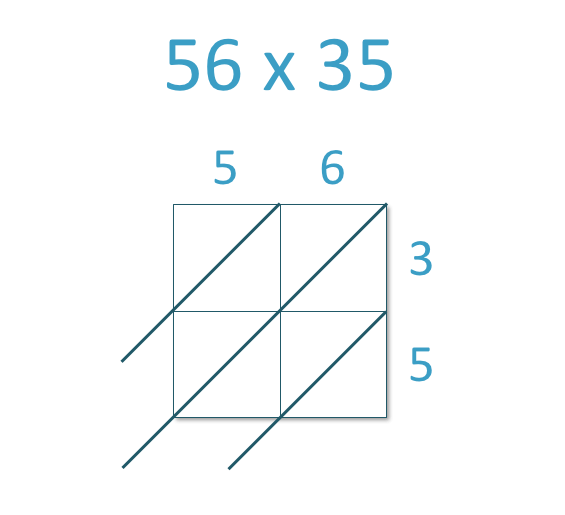Next, we multiply each of the digits of 56 by each of the digits of 35. We write the answer to each multiplication in the corresponding square.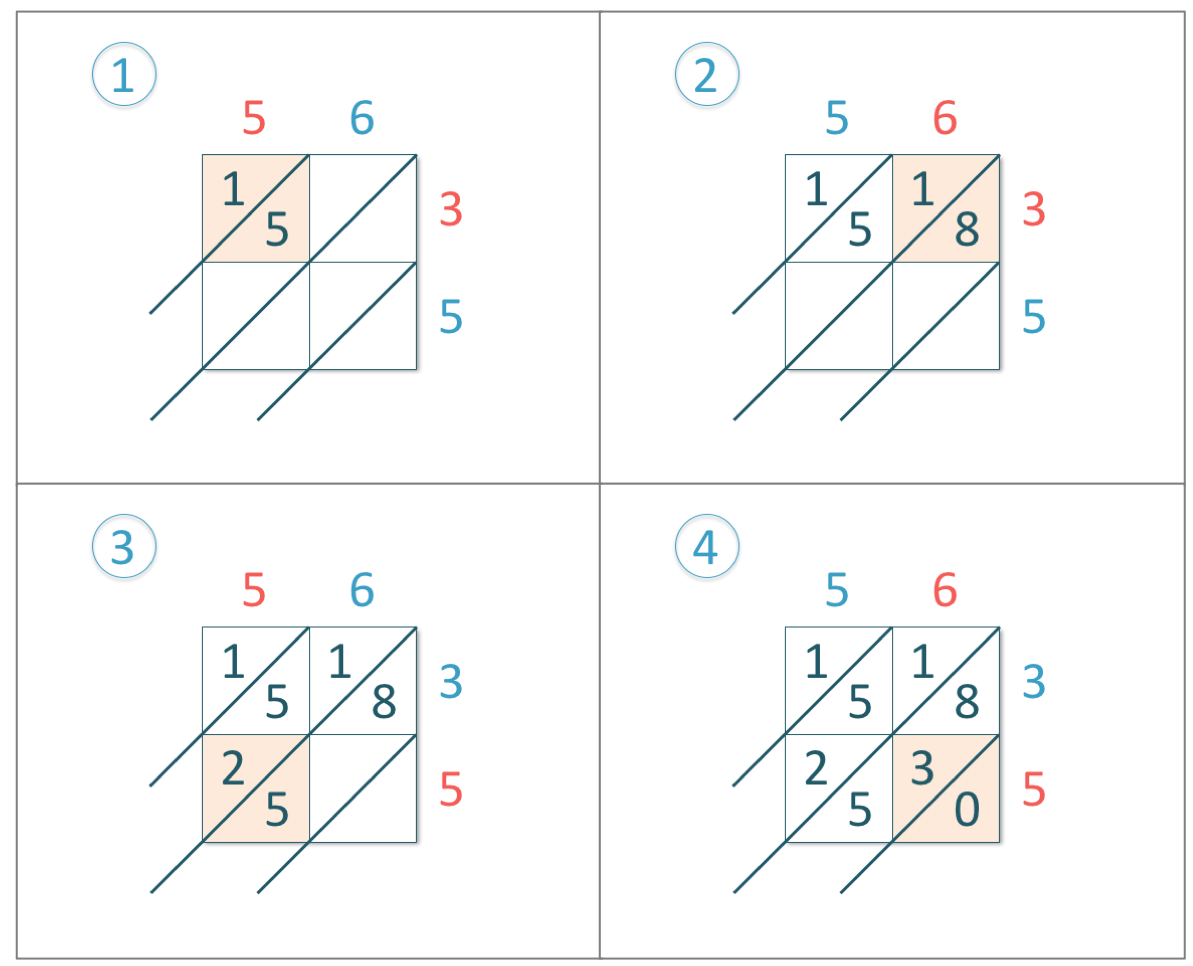Remember that we write the tens of each answer on the left of the diagonal in each box and the units of each answer on the right of the diagonal.

1) 5 x 3 = 15

2) 6 x 3 = 18

3) 5 x 5 = 25

4) 6 x 5 = 30

Now that all of the lattice squares have been completed, we add the diagonals, working from right to left.1) In the first diagonal, we have just zero. So, we write ‘0’ below.

2) In the next diagonal, we have five, three and eight.

5 + 3 + 8 = 16

So, we write ‘6’ in this diagonal and carry the 1 over to the next diagonal.

3) In the next diagonal, we have two, five, one and the one that we carried.

2 + 5 + 1 + 1 = 9

So, we write ‘9’.

4) In the final diagonal, we have one. So, we write ‘1’.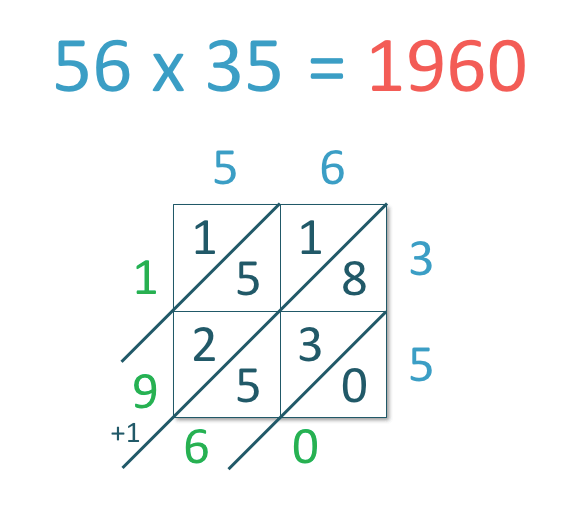We read the totals from left to right and the digits that we have are 1, 9, 6 and 0.

So, 56 x 35 = 1960

Lattice Multiplication Example 3: 86 x 67

We begin by arranging the digits of 86 and 67 as shown in the image below, with 86 written above the lattice and 67 written to the right of the lattice.Next, we multiply each of the digits of 86 by each of the digits of 67. We write the answer to each multiplication in the corresponding square.1) 8 x 6 = 48

2) 6 x 6 = 36

3) 8 x 7 = 56

4) 6 x 7 = 42

Now that we have multiplied all numbers in the lattice, we will add the numbers in the diagonals starting from the bottom right diagonal.1) In the first diagonal, we have two. So, we write ‘2’ below.

2) In the next diagonal, we have six, four and six.

6 + 4 + 6 = 16

So, we write ‘6’ in this diagonal and carry the 1 over to the next diagonal.

3) In the next diagonal, we have five, eight, three and the one that we carried.

5 + 8 + 3 + 1 = 17

So, we write ‘7’ in this diagonal and carry the 1 over to the next diagonal.

4) In the final diagonal, we have four and the one that we carried.

4 + 1 = 5

So, we write ‘5’.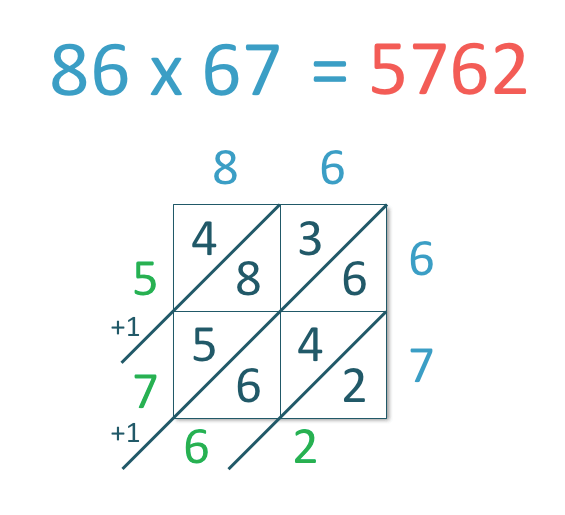In the lattice multiplication method, we read the totals from left to right and the digits that we have are 5, 7, 6 and 2.

So, 86 x 67 = 5762

The lattice multiplication method is sometimes known as the Chinese method or Gelosia multiplication.

These methods are different names for the lattice multiplication method learnt above and you can print blank lattice method grids which are also available to download above for further practice of this topic.Now try our lesson on Grid Method Multiplication where we learn how to use the grid method to multiply 2-digit numbers.error: Content is protected !!